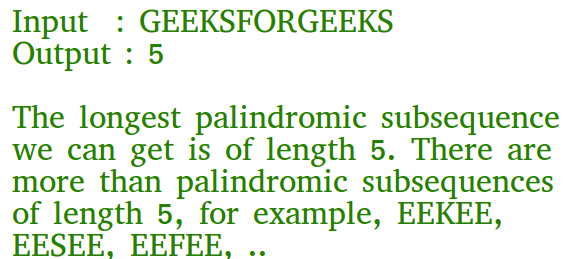# C Program for Longest Palindromic Subsequence | DP-12

• Last Updated : 13 Aug, 2021

Given a sequence, find the length of the longest palindromic subsequence in it.We provide nothing but the best curated videos and practice problems for our students. Check out the C Foundation Course and master the C language from basic to advanced level. Wait no more, start learning today!

As another example, if the given sequence is “BBABCBCAB”, then the output should be 7 as “BABCBAB” is the longest palindromic subsequence in it. “BBBBB” and “BBCBB” are also palindromic subsequences of the given sequence, but not the longest ones.

1) Optimal Substructure:
Let X[0..n-1] be the input sequence of length n and L(0, n-1) be the length of the longest palindromic subsequence of X[0..n-1].
If last and first characters of X are same, then L(0, n-1) = L(1, n-2) + 2.
Else L(0, n-1) = MAX (L(1, n-1), L(0, n-2)).

Following is a general recursive solution with all cases handled.

## C

 `// C program of above approach` `#include ` `#include `   `// A utility function to get max of two integers` `int` `max(``int` `x, ``int` `y) { ``return` `(x > y) ? x : y; }`   `// Returns the length of the longest palindromic subsequence in seq` `int` `lps(``char``* seq, ``int` `i, ``int` `j)` `{` `    ``// Base Case 1: If there is only 1 character` `    ``if` `(i == j)` `        ``return` `1;`   `    ``// Base Case 2: If there are only 2 characters and both are same` `    ``if` `(seq[i] == seq[j] && i + 1 == j)` `        ``return` `2;`   `    ``// If the first and last characters match` `    ``if` `(seq[i] == seq[j])` `        ``return` `lps(seq, i + 1, j - 1) + 2;`   `    ``// If the first and last characters do not match` `    ``return` `max(lps(seq, i, j - 1), lps(seq, i + 1, j));` `}`   `/* Driver program to test above functions */` `int` `main()` `{` `    ``char` `seq[] = ``"GEEKSFORGEEKS"``;` `    ``int` `n = ``strlen``(seq);` `    ``printf``(``"The length of the LPS is %d"``, lps(seq, 0, n - 1));` `    ``getchar``();` `    ``return` `0;` `}`

Output:

`The length of the LPS is 5`

Dynamic Programming Solution

## C

 `// A Dynamic Programming based C program for LPS problem` `// Returns the length of the longest palindromic subsequence in seq` `#include ` `#include `   `// A utility function to get max of two integers` `int` `max(``int` `x, ``int` `y) { ``return` `(x > y) ? x : y; }`   `// Returns the length of the longest palindromic subsequence in seq` `int` `lps(``char``* str)` `{` `    ``int` `n = ``strlen``(str);` `    ``int` `i, j, cl;` `    ``int` `L[n][n]; ``// Create a table to store results of subproblems`   `    ``// Strings of length 1 are palindrome of length 1` `    ``for` `(i = 0; i < n; i++)` `        ``L[i][i] = 1;`   `    ``// Build the table. Note that the lower diagonal values of table are` `    ``// useless and not filled in the process. The values are filled in a` `    ``// manner similar to Matrix Chain Multiplication DP solution (See` `    ``// https:// www.geeksforgeeks.org/matrix-chain-multiplication-dp-8/). cl is length of` `    ``// substring` `    ``for` `(cl = 2; cl <= n; cl++) {` `        ``for` `(i = 0; i < n - cl + 1; i++) {` `            ``j = i + cl - 1;` `            ``if` `(str[i] == str[j] && cl == 2)` `                ``L[i][j] = 2;` `            ``else` `if` `(str[i] == str[j])` `                ``L[i][j] = L[i + 1][j - 1] + 2;` `            ``else` `                ``L[i][j] = max(L[i][j - 1], L[i + 1][j]);` `        ``}` `    ``}`   `    ``return` `L[n - 1];` `}`   `/* Driver program to test above functions */` `int` `main()` `{` `    ``char` `seq[] = ``"GEEKS FOR GEEKS"``;` `    ``int` `n = ``strlen``(seq);` `    ``printf``(``"The length of the LPS is %d"``, lps(seq));` `    ``getchar``();` `    ``return` `0;` `}`

Output

`The length of the LPS is 7`

Please refer complete article on Longest Palindromic Subsequence | DP-12 for more details!

My Personal Notes arrow_drop_up
Recommended Articles
Page :